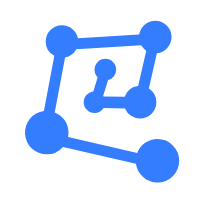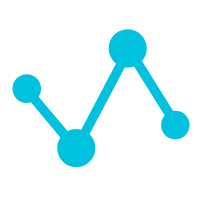# Community

Blog Events Webinars Tutorials Forum
×
Community Blog An Introduction to the Machine Learning Algorithms of the PostgreSQL MADlib Graph

# An Introduction to the Machine Learning Algorithms of the PostgreSQL MADlib Graph

This short article introduces the Machine Learning Algorithms of the PostgreSQL MADlib Graph.

By digoal

## Background

The supported algorithms are listed below:

1.  Find all shortest point-to-point paths in a given graph

3.  Depth-first

4.  The HITS algorithm calculates the hub score and authority score of each point. For example, on a web application, calculate the number of times a page is referenced, count the jumps to other pages from one page, and determine whether a page is authoritative or not.

According to the HITS algorithm, after a user enters a keyword, the algorithm calculates two values for the returned matching page: hub score and authority score. The two scores depend on (and are affected by) each other. The hub score refers to the sum of authority scores of all the exported links on the page. The authority score is the sum of the hub scores from all the imported links on the page.

5.  Find the average value of all shortest point-to-point paths in a given graph and judge the intimacy of the global relationship of all points, such as the intimacy of employees in a company and the intimacy of members in a community

6.  Find the number of reachable points for each point, the reciprocal of the distance sum of the shortest paths to all reachable points, the reciprocal of the distance average, and the sum of the distance reciprocal of all shortest paths in a given graph

It can be used to find hub points and judge whether a person has sales potential.

7.  Find the longest one among all the shortest paths in a given graph. In other words, find the two points with the worst intimacy.

8.  Calculate the degree of a specified point in a given graph: the number of outbound sides and inbound sides.

It can be used to judge whether the point is a hub.

9.  Use the PageRank algorithm to output a probability distribution in a given graph, indicating the probability of a person to reach any specific vertex traversing the graph. This algorithm was initially used by Google to rank websites. The World Wide Web was modeled as a directed graph with vertices representing websites. The PageRank algorithm was originally proposed by Larry Page and Sergey Brin.

10.  Find the shortest path

Given a graph and a source vertex: The single-source shortest path (SSSP) algorithm finds a path from the source vertex to all the other vertices in the graph, making the sum of weight values of path sides minimum.

11.  Find the weakly connected component (WCC) in a given directed graph. WCC is a subgraph of the original graph, and all its vertices are connected to each other through some path, ignoring the direction of sides. For an undirected graph, weakly connected components are also strongly connected components. This module also includes many auxiliary functions that operate on the WCC output.

A graph may be split into multiple paths. The points in the paths are non-repetitive and non-overlapping. So, the graph is a combination of some paths.

Component ID (component_id) is the ID of the first vertex of the group, which is the start point ID. Therefore, the component ID is disconnected and discontinuous.

For example:

``````INSERT INTO vertex VALUES
(0),
(1),
(2),
(3),
(4),
(5),
(6),
(10),
(11),
(12),
(13),
(14),
(15),
(16);
INSERT INTO edge VALUES
(0, 1, 1),
(0, 2, 1),
(1, 2, 1),
(1, 3, 1),
(2, 3, 1),
(2, 5, 1),
(2, 6, 1),
(3, 0, 1),
(5, 6, 1),
(6, 3, 1),
(10, 11, 2),
(10, 12, 2),
(11, 12, 2),
(11, 13, 2),
(12, 13, 2),
(13, 10, 2),
(15, 16, 2),
(15, 14, 2);  ``````

The components are listed below:

``````0,1,2,3,5,6
4
10,11,12,13
14,15,16

id | component_id
----+--------------
0 |            0
1 |            0
2 |            0
3 |            0
5 |            0
6 |            0
4 |            4
10 |           10
11 |           10
12 |           10
13 |           10
14 |           14
15 |           14
16 |           14
(14 rows)  ``````

0 0 0
Share on

# Related Products

• ##Database Overview

Fully managed and less trouble database services

• ##AnalyticDB for PostgreSQL

An online MPP warehousing service based on the Greenplum Database open source program

• ##Machine Learning Platform For AI

An end-to-end platform that provides various machine learning algorithms to meet your data mining and analysis requirements.

• ##Machine Translation

Relying on Alibaba's leading natural language processing and deep learning technology.